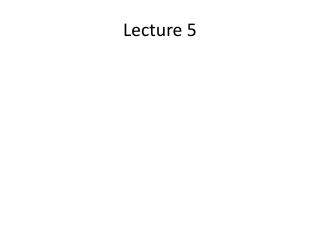# Lecture 5 - PowerPoint PPT PresentationDownload PresentationLecture 5

Lecture 5Download Presentation## Lecture 5

- - - - - - - - - - - - - - - - - - - - - - - - - - - E N D - - - - - - - - - - - - - - - - - - - - - - - - - - -
##### Presentation Transcript

1. Lecture 5

2. Indifference Curve • Indifference Curve (IC): It shows the combination of goods or services that yields the same level of utility. • A consumer can purchase different combination of goods and services. Suppose he consumes two goods. We can construct different bundles with different combination of these two goods. We will conclude that two different bundles are in the same indifference curve if the consumer derives same level of satisfaction from these two bundles.

3. Example: Consider two goods X and Y. Now let us construct two bundles, bundle A= (X1, Y1) and bundle B= (X2, Y2) where X1 indicates the amount of good X and Y1 indicates the amount of good Y. Now if a consumer derives same level of satisfaction from both of the bundle then we conclude that the bundles are in the same IC. By connecting the bundles that gives the same level of utility we get the indifference curve.

4. Shift of Indifference CurveAn increase in utility is shown by a rightward shift of IC and a decrease in utility is shown by a leftward shift of IC. So in the figure IC3 shows higher utility than IC1 and IC2 shows lower utility than IC1.

5. MRS ( Marginal Rate of Substitution) • The slope of the indifference curve is known as MRS. It shows at what rate a consumer is willing to substitute one good for another good. • MRS= Slope of IC= ΔY/ΔX • So the Marginal Rate of Substitution shows that if we increase the quantity of good X ( by ΔX) then we need to decrease the quantity of good Y ( by ΔY) to keep the consumer’s utility constant.

6. Budget Constraint • Individuals have limited income or resource. That’s why we can’t consume unlimited amount of goods and services. The constraint in our income or budget is shown by budget line. • Budget Line: The budget line shows the combination of goods or services that a consumer can purchase given his limited income. • It is a downward sloping straight line. Suppose M= income X = amount of good X Px= Price of good X Y= amount of good Y Py= Price of good Y So the equation of budget line is Px*X + Py*Y= M

7. The slope of the budget line is the price ratio of the goods. Why? So the equation of budget line is Px*X + Py*Y= M Rearrange the equation Py*Y= M- Px*X Y= M/Py- (Px/Py)*X In this equation • intercept is M/Py • slope is Px/Py where Px/Py is the price ratio of the good X and Y. • So we can conclude that the slope of the budget line is the price ratio of the two goods.

8. How to find utility maximizing bundle/ optimal bundle • A consumer if better off if he can reach to a higher indifference curve. • Due to the limited resource he has to be on the budget line. So our purpose is to find a combination/bundle of goods on the budget line that allows us to attain maximum utility. This bundle of goods is known as optimal bundle

9. Necessary Condition of Utility Maximization • Tangency Condition: Utility will be maximized if the budget line is tangent to IC. For any bundle where budget line cuts the IC will not be an optimal solution because there is a way to improve the utility by moving to higher IC. • Tangency condition implies that the slope of IC and budget line are same. • Slope of IC= MRS • Slope of budget line= Price Ratio= Px/Py • So the tangency condition of utility maximization is MRS= Px/Py So A is the optimal bundle that is the solution to the utility maximization